An introduction to Genetic Network Programming (GNP） and its coding procedure

<Now revising English sentences>

[Japanese]

Evolutionary computation is based on the mechanism of evolution of living things and applied to many optimization problems, program making etc.
Typical evolutionary computations are:

• Genetic Algorithm, GA ,
• Genetic Programming, GP .

GA and GP mainly represent solutions using string and tree structures, respectively.
Genetic Network Programming (GNP）[3,4] has been proposed as a graph-based evolutionary computation, which is an extension of GA and GP, and also based on the idea that a graph structure has distinguished abilities to represent programs because a brain in a living thing is composed of a network structure.

In this website, the basic strucutre of GNP and how to make program code in C language are introduced.

Template of GNP code　ver. 1.0.1 (bug modification 08/07/14)
This is a sample code of GNP which is based on the explanation below.
There are some differences between the sample code and the following explanation, e.g., variable names, but the functions are the same.

The strucutre of GNP and its features

Component: Node

1. A program of GNP consists of Judgment node （Fig. 1(a)）and Processing node（Fig. 2(b)）. For example, a judgment node has branch decision functions using sensor inputs, and Processing nodes decises an action to be executed.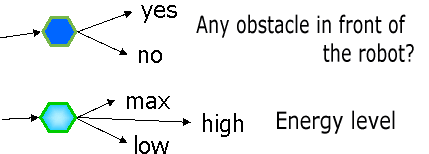(a) Judgment node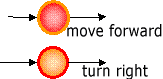(b) Processing node Fig. 1 An example of nodes

How to represent node information: Gene strucuture

2. Information of each node is represented by the gene structure (array). *1

 NT ID d CA CB CC

Fig. 2 Gene structure (array)

The gene structure corresponds to the following figure (Fig. 3).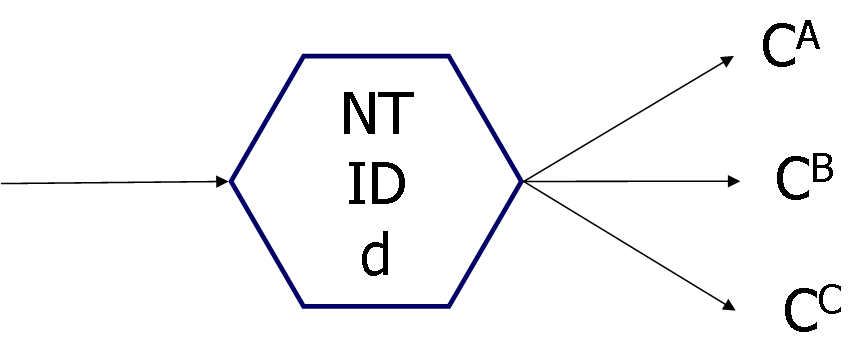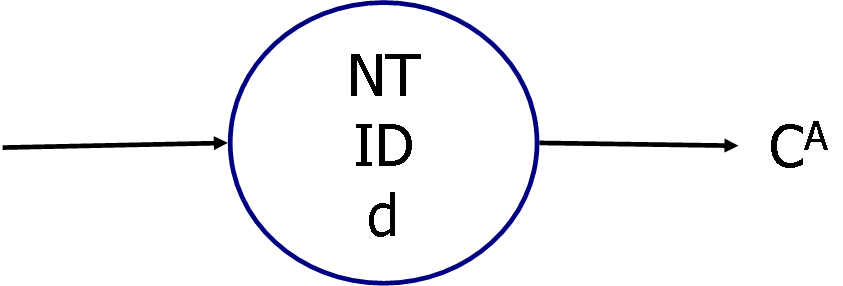Fig. 3 Correspondence between gene and node

NT：node type (1: Judgment node, 2: Processing node）
ID： node function

In Fig. 1, for example,

NT=1 (Judgment node) and ID=1 shows a judgment node which judges "If there is any obstacle in front of the robot or not".
NT=1 (Judgment node) and ID=2 shows a judgment node which judges "remaining energy level".
NT=2 (Processing node） and ID=1 shows a processing node deciding "move forward" action.
NT=2 (Processing node) and ID=2 shows a processing node deciding "turn right" action.

d: Time delay needed for node execution, which can be used to consider the time spend on the judgment/processing. Other function of time delay is to avoid judgent loop, which is actually very important function.

The setting of time delay depends on a problem. For example,

Time delay d of judgment node = 1
Time delay d of processing node = 5

CA, CB, CC: Node numbers connected from this node. When the total number of nodes in GNP individual is "N", each node has a unique number from 0 to N-1. Therefore, if CA=15, there is a connection to node number 15.

In the case of judgment node, if the judgment result is "A", the current node is transferred to node CA. If the judgment result is "B", then next node number is CB. The node judging energy level in Fig. (a), for example, if the energy is max, next node is CA; if high, next node is CB; if low, the next node is CC.

Processing node has no branch decision function, therefore, after executing an processing function, e.g., "move forward", "turn right", etc., the current node is transferred to CA. (CB and CC are not used)

Gene information of one node is represented by the following strucutre in C language.

 struct gene_structure{  int node_type;  int node_func;  int node_delay;  int connect[NUM_BRANCH]; } /* NUM_BRANCH: max number of branches (connections)*/

*１ In [3,4], there are time delay on node transition, but until now, it has not been used. Therefore, it is omitted in this website.

Directed graph generation

１．Declaration of node variables

GNP program is composed of a large number of judgment nodes and processing nodes that are connected as a network structure shown in Fig. 3.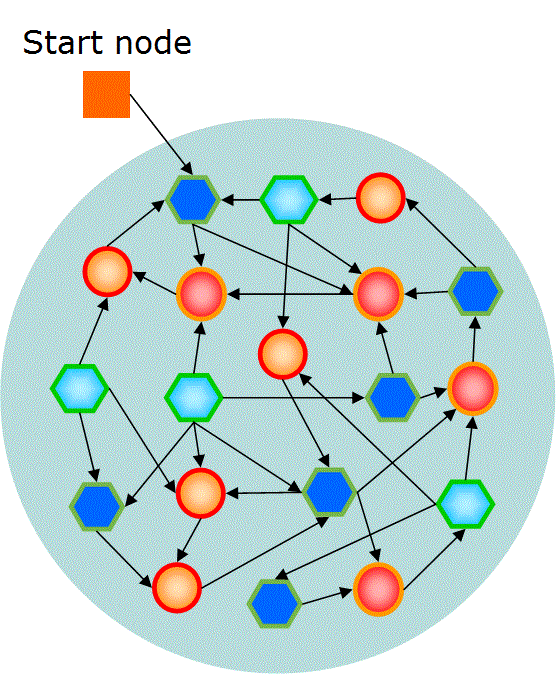Fig. 3 Basic structure of GNP

First, a variable of a start node information is declared. The start node shows the number of node that is firstly executed in the program.

int initial_node;

Next, we set the number of judgment nodes and processing nodes, which is defined as NODE_SUM. The number of nodes is set depending on the complexity of the problem. For example, if there are four kinds of nodes as Fig. 1, totally 20 nodes are parepared as a first setting, supporsing that five nodes per each kind node function is used. In this case, the gene structure of "NODE_SUM nodes" can be declared by

struct gene_structure gene[NODE_SUM];

Since GNP is an evolutionaty computation, many individuals have to be prepared. Therefore, gene strucutres for all the individuals (the number of individuals is " IND")a are prepared as follows.

 int initial_node[IND]; /* start node numbers for all the individuals */ struct gene_structure gene[IND][NODE_SUM]; /* Judgment nodes and Processing nodes for all the individuals */

2. Initialization of the individuals

To create individuals in the first generation, all the variables of nodes are initialized. Node type (judgement/processing) and Node functions are randomly assigned, time delay is set at arbitrary values, and node connections are randomly determined. Suppose that one start node and NODE_SUM nodes including judgment/processing nodes are used, then all the nodes are initialized as follows.

 for (int ind; ind

The above program initializes all the nodes and "IND" structures of individuals are created.

*2　Judgment node numbers are from 0 to JUDGE_SUM-1. Processing node numbers are from JUDGE_SUM to NODE_SUM-1.

*3　In the above program, node functions are randomly assigned, but a large degree of freedom often causes difficulties of search for good solutions. Therefore, assigning the fixed number of node functions often shows better performance. In Fig. 1, for example, since there are four kinds of judgment/processing nodes, totally 20 nodes are used preparing five nodes per each kind. The best number of nodes shoudl be determined considering the complexity of the problem, and how to find the optimal number is a remaining problem.

Program execution (node transition)

How to execute individual (number ind （0<=ind<IND, IND: the number of individuals) ) is introduced.

1. A varialbe showing the "current node number" is declared.

int current;

2. Store the first node number according to the start node information.

current=initial_node[ind];

3. Transfer to the next node after executing the function of judgment/processing node.

The program code considering the above is described as follows.

 int current; int judge_result; for (int ind; ind

Genetic operators

Crossover and mutation are used in GNP.
In the previous research, crossover and mutation are not applied to the same individuals to avoid destructive changes of the structures, but the number of individuals created by crossover and those created by mutation are determined in advance. For example, 120 individuals are created by crossover and 180 indiiduals are created by mutation.

Crossover

1. Select two individuals (parents) using arbitrary selection method according to the fitness
2. From node number 1 to N-1, each node is selected as a crossover node with the probability of Pc.
3. Selected nodes are exchanged between two individuals. For example, node 5 is selected as a crossover node, the gene information of "node 5 in parent1" and that of "node 5 in parent 2" are exchanged.

 for (int node_num=0; node_num

* The above code of crossover is a little different from the sample program. But, the function is the same.

Mutation

1. Select one individual using arbitrary selection method according to the fitness

Mutation of node connections:
2. Each branch (connection) is independently selected as a mutation branch with the probability of Pm.
3. Selected brandh is re-connected to other node.

Mutation of node functions:
4. Each node is independently selected as a mutation node with the probability of Pm.
5. The functions of selected mutation nodes are randomly changed. However, processing node is not changed to judgment node, and vice versa.

 for (int node_num=0; node_num

*4 In the above source code, some redundant loop occurs in the mutation of connections of Processing nodes which uses only one connection. The code is described as simple as possible for easy understanding.

References

 J. H. Holland: Adaptation in Natural and Artificial Systems, University of Michigan Press, 1975
 J. R. Koza: Genetic Programming: On the Programming of Computers by Means of Natural Selection, MIT Press. 1992
 S. Mabu, K. Hirasawa and J. Hu: A graph-based evolutionary algorithm: Genetic Network Programming (GNP) and its extension using reinforcement learning, Evolutionary Computation, Vol. 15, No. 3, pp. 369-398, 2007
 間普 真吾，平澤宏太郎: 遺伝的ネットワークプログラミングのアーキテクチャについて，システム制御情報学会誌, システム／制御／情報，Vol. 55, No. 11, pp. 480-485, 2011(in Japanese)